# Polygons and Handshakes

We’ll spend the next couple weeks looking at various counting problems. This topic, called combinatorics, is often studied along with probability, but many of the topics we’ll see here feel more like geometry problems! Here, we’ll be counting the diagonals of a polygon, and handshakes between people at a party.

## Counting diagonals

Counting Diagonals

How many diagonals can be drawn for a polygon with 15 sides? How many diagonals can be drawn for a polygon with n sides?

I tried to find a pattern in how many diagonals there are for a polygon with 4 sides, 5 sides, etc., but I couldn't extract a pattern. Please show me a method for this question.

Here are examples of polygons with smaller numbers of sides, showing sides in blue and diagonals in red: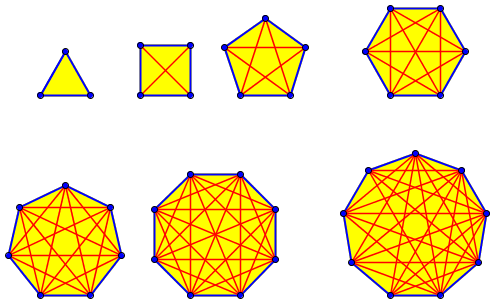Molly probably didn’t go as far as 9 sides; but as I count here, for n = 3, 4, 5, 6, 7, 8, 9, the number of diagonals is 0, 2, 5, 9, 14, 20, 27. If you made a table of these numbers and apply some of the methods we’ve discussed previously for finding patterns in sequences, you could probably find one – but you wouldn’t be sure it’s the right pattern, and might not find a formula. (By the way, my pictures are all of regular polygons, but nothing will change if we make them irregular, except that if the polygon were not convex, some diagonals might go outside. The counts wouldn’t change.)

Doctor Wilkinson answered, starting with Molly’s very appropriate plan, counting and then looking for patterns:

Looking for a pattern with polygons of 4 sides, 5 sides, etc. was an excellent idea. Sometimes, though, it's pretty hard to see a pattern in a sequence of numbers. It may be better to look for the pattern in the whole process of counting the diagonals. That is, look for a systematic way of counting diagonals as you look at specific cases like 4 and 5.

So let's take another look at polygons with 4 and 5 sides.

For 4 sides, we have a quadrilateral ABCD.  The diagonals are:

AC
BD

There's one diagonal containing A, one containing B, one containing C, and one containing D.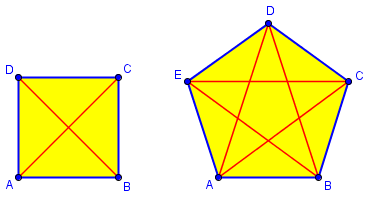We’re looking not just for the numbers, but for a way to count that will lead to a formula. So we haven’t even counted the two diagonals yet, but just noticed that there is one from each vertex …

Let's leave it at that for a minute, and look at case 5.

Here we have a pentagon ABCDE.  The diagonals are:

AC
BD
BE
CE

Here we have two diagonals containing A, two containing B, two containing C, two containing D, and two containing E.

This way of describing it suggests the beginning of a pattern!

So for 4 sides there is one diagonal for each vertex and for 5 there are two. Why? Well, there is a diagonal from each vertex to each other vertex except the two adjacent vertices. For 4 vertices, there are three other vertices for each vertex, and two of them are adjacent, which leaves 1; for 5 vertices, there are four other vertices for each vertex, and two of them are adjacent, which leaves 2.

So for n vertices, there will be n – 3 diagonals through each.

Okay now, we're starting to get somewhere. For four vertices there is one diagonal containing each vertex, but we don't end up with four diagonals but just two. For five vertices there are two diagonals containing each vertex, but we don't end up with ten diagonals but just five. What's going on?

Aha!  If you count one diagonal for every vertex of the quadrilateral, you're counting every diagonal twice, because every diagonal contains two vertices. And similarly for the pentagon. So you have to divide by two.

I hope this helps you with the general problem.

Without stating the actual answer, he has effectively given it, so that Molly should be able to write the formula now: $$Diagonals = \frac{n(n-3)}{2}$$ Applying this to small values of n, you should find the numbers I got above. But to be honest, when I wrote those numbers down, I wasn’t using this formula; I used the recursive formula we’ll see below, with which I could write each number based on the previous one. And then I checked that the actual count matched by actually seeing, for instance, that with n = 9, there were 6 lines coming from each of 9 vertices, for a total of $$\displaystyle\frac{(6)(9)}{2} = 27$$.

Now, what do you get when n = 15? I’m not about to draw and count for that case!

## Explicit and recursive formulas

A student in 1997 had been shown the formula that results from this thinking, and wanted more:

Diagonals of Polygons

My teacher showed us how to find diagonals using two different equations. I lost the sheet which I wrote them on. Do you know them? One of them might have been n(n-3)/2 and I am not sure of the other one, but it was called recursive.

Doctor Wilkinson answered this one too, first correcting the implied question (since finding diagonals would mean actually drawing or naming them), and quickly deriving the formula:

The question apparently is "How many diagonals does a polygon with n sides have?"

You have remembered the first formula correctly: it is n(n-3)/2.  One way to see this is to notice that you can draw (n-3) diagonals from every vertex of the polygon. This is because there are (n-1) other vertices, but two of them are adjacent vertices and so don't count towards making diagonals. This seems to give n(n-3) diagonals, but this way of counting counts every diagonal twice since each diagonal connects to two vertices, so you have to divide by 2.

This, of course, is what we just did, stated fully.

By a recursive formula, we mean a way of expressing the answer for n vertices in terms of the answer for n-1 vertices. Suppose, for example, that you already know the answer for a polygon with n-1 vertices. Now if you add another vertex between two of the vertices of the original polygon, then all the diagonals of the original polygon will still be diagonals of the new polygon, and so will the side joining the two vertices that you added a new vertex between, and so will the line segments joining the new vertex to all the other vertices of the original polygon. (Got all that?) So if we let diag(n) be the number of diagonals for a polygon with n sides, we get the formula:

diag(n) = diag(n-1) + n - 3 + 1 or

diag(n-1) + n - 2

Here (for n = 6) we insert a new vertex into a pentagon, which adds 3 new diagonals and changes one side to a diagonal (all in purple):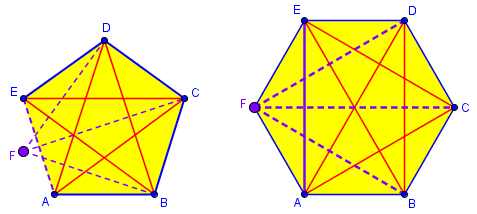In applying this method to make my list above, I first knew that for n = 5, Diag = 5; then for n = 6, I had to add 4 to that (6 – 2 = 4); each time, I added a number greater by 1 than the last one.

The first formula is better, since it actually gives you the answer.  But sometimes it's easier to get a recursive formula first and use that to get an explicit formula (your first formula is an explicit one since you only need the number of vertices in the polygon to get the number of diagonals in that polygon).  This is called "solving the recursion."  Sometimes a recursive formula is the best you can do because there simply is no explicit formula.

We’ll be seeing below another way to think of it, summing a series; this is closely related to the recursive formula, as for example my count for n = 6 can be written as 2 + 3 + 4. But doing this all the way to n = 15 (or 42, or 100, as in other questions) would not be pleasant.

## Handshakes all around: Three ways to get the formula

Our next question, from 2001, will help us move over to a different but related problem:

Handshakes and Polygon Diagonals

Dear Dr. Math,

I can't figure this one out!

If a polygon has 42 sides, how many diagonals does it have?

That’s a lot of sides; to answer, you’ll need some sort of formula.

Doctor Pete answered, starting with some leading questions like those we’ve seen:

Let's look at a hexagon, with 6 sides. Pick a vertex. How many diagonals come from that vertex? (Three.) How many vertices in a hexagon? (Six.)

So there should be 3*6 = 18 diagonals, but in fact if you count them there are only half as many. Why? Well, since a single diagonal joins exactly two vertices, for each vertex we counted the same diagonal twice.


Each diagonal is the starting point for 3 “directed diagonals”: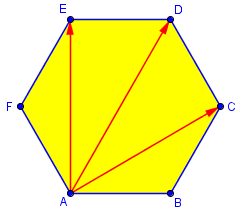Two of these correspond to one actual diagonal.

So look at a 42-gon.

How many diagonals come from a single vertex?
How many vertices are there in a 42-gon?
How many diagonals are in a 42-gon?

A diagonal can go to any vertex except the one it starts at and the two neighbors, so there are 39 destinations for each of the 42 starting points. This gives a total of $$42\cdot39 = 1638$$ “directed diagonals”; dividing by 2, we have 819 diagonals. We didn’t need an actual formula after all, did we?

Now Doctor Pete switched to a non-geometrical problem, which we’ll be focusing on for most of the rest of this post.

### Handshakes per person

Let's look at something similar to this. If you have 100 people at a party, and everyone shakes hands with everyone else, how many handshakes take place? Well, pick a person. He shakes hands with 99 others. In fact, each person shakes hands with 99 others, so there should be 100*99 = 9900 handshakes; but again, it takes two people to shake hands, so this is double the correct amount, which is 4950 handshakes.

By now the pattern should be clear. If there are n people at a party, the number of handshakes is n(n-1)/2.

This is like counting, not the diagonals only, but the diagonals together with the edges of a polygon – all the lines joining any two vertices. This time, there are n – 1 handshakes/segments for each person/vertex. Here are the handshakes originating from one person in a six-person party: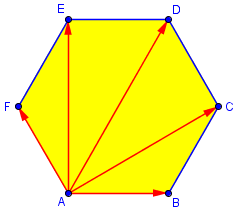### Summing a series

Now, there is a different way to count these handshakes. The first person shakes hands with 99 others. The second person shakes hands with 98 others, not including the first person, whom we have already counted. The third person shakes hands with 97 others, not including the first two. And so on, until the 99th person, who shakes hands with the 100th person. So there are

99 + 98 + 97 + ... + 2 + 1

handshakes. But we have seen that this is equal to 100*99/2, and this suggests a formula. In general, if there are n people at a party, then counting the number of handshakes in two different ways gives the formula

1 +  2 + ... + (n-2) + (n-1) = n(n-1)/2.

Such a number is called a triangular number, and the formula is known. We have just proved it, merely by seeing our handshake number from a different perspective. In a moment we’ll see how to find it if we hadn’t already known the formula.

Here is a representation of the 10 edges of a pentagon as the sum 4 + 3 + 2 + 1, starting at A, B, C, and D respectively: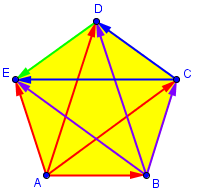The diagonals can similarly be seen as a sum, but it’s harder to see without thinking in terms of the recursive formula I showed above.

Finally, we can prove this summation in yet another way. Write

S =     1 +     2 + ... + (n-2) + (n-1)
S = (n-1) + (n-2) + ... +     2 +     1
----------------------------------------
2S =     n +     n + ... +     n +     n,

and since there are n-1 "n"'s, then we have 2S = n(n-1), or

S = n(n-1)/2.

This is how we prove the formula for triangular numbers, or equivalently for the sum of an arithmetic series.

### Another way: counting pairs

I couldn’t find am archived page where we explicitly mentioned this, but the total number of segments can lso be thought of as the number of pairs of vertices, which is the number of combinations of n vertices taken two at a time, written as $${{n}\choose {2}} = _nC_2 = \frac{n!}{2!(n-2)!} = \frac{n(n-1)}{2}$$

### Back to the diagonals

However, the handshake analogy isn't exact.  At a party, each person gets to shake hands with everyone but himself.  But in a polygon, each vertex doesn't get to form a diagonal with himself... or with the two vertices nearest him.  (He's already connected to them by edges.)  So for a polygon, we have to subtract the number of edges from the total for the handshake problem:

S = n(n-1)/2 - n

= [n(n-1) - 2n]/2

= [n(n - 1 - 2)]/2

= n(n-3)/2

$$Diagonals = Segments – Edges = \frac{n(n-1)}{2} – n = \frac{n^2-n-2n}{2} = \frac{n^2-3n}{2} = \frac{n(n-3)}{2}$$

## The Handshake Problem on its own

The handshake question is one we have often been asked on its own, so let’s look at a couple answers to that, with or without reference to polygons. First, one from 1997:

Handshake Problem

Our 5th grade math class was learning to solve story problems by looking for a pattern and setting up a chart. Most of the problems were of the nature: If you had 8 people in a group and each one had to shake everyone else's hand one, how many handshakes would take place?

After working through several problems, one boy in the room said "I can get it faster without making a chart. If you multiply whatever the number is by one less, then divide by 2, you get the right answer each time."

Will this always work?  Why?

Yes, it will always work.  The boy concerned was very observant.  A good way to model the situation is to think of an n-sided polygon, which has n vertices.

This time the question was about handshakes, but the answer is the polygon model. It works both ways! He uses the lines-per-vertex approach:

Now consider how many diagonals you can draw between the vertices, and also include two lines connecting a particular vertex to the two adjacent ones. It is clear that there are (n-1) lines joining any one vertex to the other vertices in the polygon. If we consider each of the n vertices, each requires (n-1) lines to join to the other vertices. There are thus n(n-1) links. But each of these links has been produced twice, once from each end, and so the number n(n-1) is too large by a factor of 2.

Hence total number of connections joining every vertex to every other one is:

n(n-1)
------
2

This is the result that the boy discovered by observation.

## Handshakes again, two ways

Finally, here is a question from 2001:

How Many Handshakes?

I'm trying to help my daughter figure out how to set up this problem:

There are 40 people in a room. They shake each other's hands once and only once. How many handshakes are there altogether?

We know that that 40 people shake hands, so each person shakes 39 hands. The first person shakes 39 hands, the second person shakes 38 hands, the third person shakes 37 hands, and so on.

How do you put this into a formula to solve the problem?

One sentence there is the start to the handshakes-per-person approach, and the next is the start to the series approach. Doctor Greenie answered by completing both, starting with the series:

Hi, Kathy -

This is a great problem for young math students to work on using two completely different approaches, so they can see that they get the same answer.

You have a good start on the first approach. The total number of handshakes is

39 + 38 + 37 + 36 + ... + 3 + 2 + 1

To find this total without having to add all these numbers together, notice the following:

The average of the first and last numbers is 20:
(39+1)/2 = 20.

The average of the second and next-to-last numbers is 20:
(38+2)/2 = 20.

If you think about it, you should be able to see that all the numbers can be paired in groups of two whose average is 20. You will have 39 numbers (1 to 39) whose average is 20 - so what is the sum of all those numbers? It is just the number of numbers multiplied by the average of the numbers: 39*20 = 780.

Next, the multiplication method, explained in a way that matches a common way to explain combinations:

Here is the other (more mathematically sophisticated) approach:

Each handshake consists of two people shaking hands. How many ways can you choose those two people if there are 40 people in the group? You can choose any one of 40 people for the first person involved in the handshake; then for each of those 40 choices, you have 39 choices for the second person. So you have 40*39 = 1560 ways of choosing the two people for the handshake.

But wait a minute. Person A shaking hands with person B is the same as person B shaking hands with person A; this means that in counting the 1560 handshakes you have actually counted each one twice, so the actual number of handshakes is half of 1560, or 780.

Two seemingly completely different ways to look at the problem, and they give the same answer!

There’s a lot of dividing by two going on around here; both methods have a lot in common.

In gathering these (and several other) discussions of these two related problems, I ran across some other “handshake” problems that are too good to skip, even though they are not about counting. They’ll be the subject of the next post, before I get back to counting.

### 3 thoughts on “Polygons and Handshakes”

This site uses Akismet to reduce spam. Learn how your comment data is processed.